### Solution to the Easter Monster Puzzle: Formal Logic and Number Pair Chains

By Bob Hanson and Dave Marans, March 12, 2008.

Shown below is the puzzle known as the Easter Monster. This page shows an interesting solution (we think) involving straightforward Medusa strong chain analysis with a twist involving a new way to define a strong chain (based on formal logic) and the synchronizing of strong chain parities to extend the chain and provide further key elimination opportunities.

### The Easter Monster puzzle

The inherent symmetry of this puzzle, particularly in the lower left four blocks (Blocks 4, 5, 7, and 8) suggests a number-pair analysis. We see, for example, 37 both in Blocks 4 and 8, and we see 46 both in blocks 5 and 7. Our solution will depend strongly upon this relationship.

Note, too, that the 3 and 7 in Block 4 are aligned vertically with the 6 and 4 in Block 7. Similarly, the 6 and 4 in Block 5 are aligned vertically with the 3 and 7 in Block 7. Also, the 3 and 7 in Block 4 are aligned horizontally with the 4 and 6 Block 5, and the same goes for the 3, 4, 6, and 7 in Blocks 7 and 8.

This is a key observation.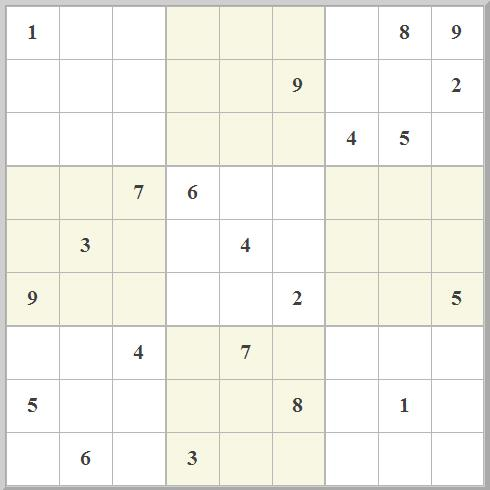(In all cases, click on the image to load that configuration into a new page.)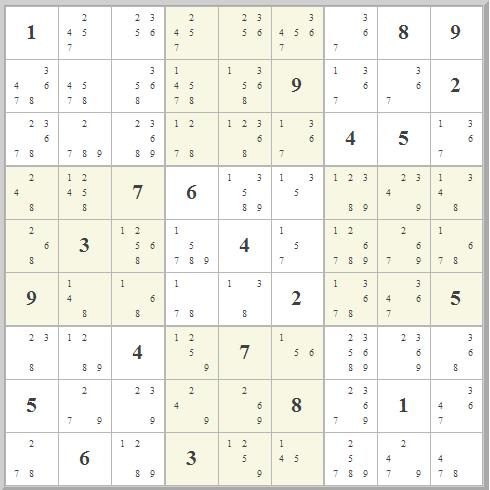The marks associated with this puzzle are shown on the right. The symmetry of the puzzle leads to four runs of cells with the interesting characteristic that each run contains only 3-value cells for which two of the possible values in each cell are the same:
• 248 268 238 278 in Column 1
• 135 157 156 154 in Column 6
• 184 186 187 184 in Row 6
• 279 239 249 269 in Row 8
A Medusa Strong Chain Analysis of this puzzle reveals just 12 short strong chains:
```Chain 1:  r2c1#4(a) r4c1#4(A)Chain 2:  r3c2#9(b) r3c3#9(B)Chain 3:  r2c7#1(c) r3c9#1(C)Chain 4:  r4c2#5(d) r5c3#5(D)Chain 5:  r4c5#9(e) r5c4#9(E)Chain 6:  r6c2#4(f) r6c8#4(F)Chain 7:  r1c6#4(g) r9c6#4(G) r8c4#4(g) r8c9#4(G)Chain 8:  r7c2#1(h) r9c3#1(H)Chain 9:  r7c1#3(i) r8c3#3(I)Chain 10:  r7c7#5(j) r9c7#5(J)Chain 11:  r8c2#7(k) r9c1#7(K)Chain 12:  r7c6#6(l) r8c5#6(L)
```
There is certainly not much to work with here!

### Initial Considerations: Number Pair Chains

Simple techniques involving naked/hidden cells, locked cells, X-wings, X-chains, Y-chains, XY-chains all provide no advance in solving this puzzle. Instead, we have to consider chains of number pairs.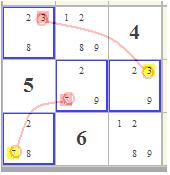Consider just Block 7, shown on the left: There are two short X-chains in this block, involving 3s and 7s (Chains 9 and 11). There are just two ways these two chains can be coupled:
 The 3 and 7 are both in the first column or both in the second row. One of the numbers (3 or 7) is in the first column and one of the numbers (7 or 3) is in the second row.

These are the only two possibilities for the Medusa strong chains involving 3 and 7 in Block 7.

We use the following logic, which we dub Frege Logic (pronounced FRE-guh, after Friedrich Ludwig Gottlob Frege, ):
```Not both (not-P and not-Q)
Not-P
Therefore Q
```
In this case P="r9c1=7" and Q="r7c1=3". So the work is to first show that "r9c1<>7 and r7c1<>3" is invalid, then show that r9c1<>7, thus proving that r7c1=3.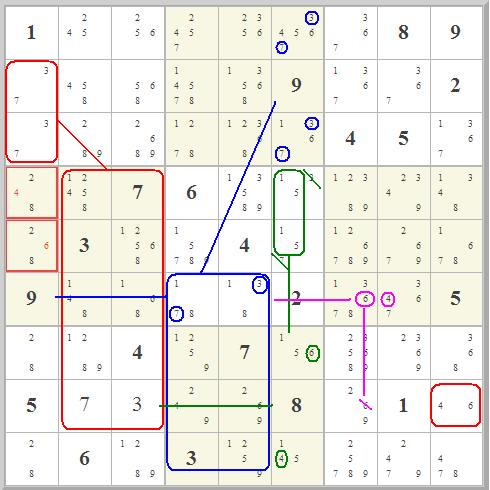First consider the effect of 3 and 7 in the same row in Block 7:
• That forces a naked 37 pair in the first column of Block 1 and a naked 28 pair in the first column of Block 7.
• That forces 4 and 6 into the first column of Block 4 and out of bottom row of that block, revealing a naked 18 pair there.
• That in turn forces 37 into the bottom row of Block 5 AND 46 into the bottom row of Block 6.
• The 37 forces a 37 naked pair in the third column of Block 2, which forces a 15 naked pair in the third column of Block 5, which forces 4 and 6 to be in the third column of Block 8, which forces 4 and 6 out of the center set of cells of Row 8. Note that r8c9 must be 4.
• The two naked pairs 18 and 37 in Row 6 force 4 and 6 into r6c8 and r6c7, respectively.
• This forces 6 (in particular) into r6c7 and out of r8c7. But that then only leaves r8c9 for 6. This is a contradiction, because we've already said that 4 must be in r8c9.

If you consider what is going on here, basically we are following chains of number pairs. The same analysis for the effect of having 3 and 7 in the first column of Block 7 proves equally impossible. You can follow the number pair chain yourself; it reveals a 29 naked pair in the center row of Block 7, which forces 4 and 6 into that row, leading up to a 46 naked pair in the third column of Block 2. Continued following of the naked pairs resulting from that leads to (in one analysis) three 18 naked pairs in Row 6.

The logical result is that if 3 and 7 cannot both not be in the first column of Block 7 and, in addition, that 3 and 7 cannot both be in that column, then one of each of the 3 and 7 must be in the first column of that block.

The result is that we have now synchronized the two strong chains in Block 7. Or, to put it another way, we have one 4-element strong chain (instead of two 2-element strong chains) in that block.

### Initial Eliminations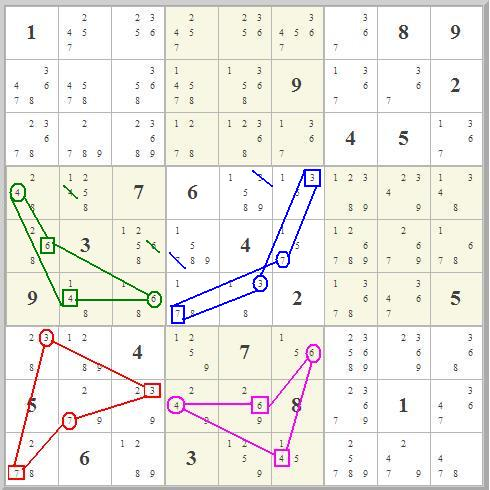The same analysis in the other three blocks in this four-block set proves valuable. We find the same result -- 4 and 6 cannot be in the same row or column in Block 4; 3 and 7 cannot both be in the same row or column in Block 5, and 4 and 6 cannot be in the same row or column in Block 8.

Note that this finding -- that exactly one of two possibilities must be X and the other must be Y -- is precisely the definition of a strong edge. (In a strong edge there are two mutually exclusive possibilities, one of which must be true. For example, a cell with only two possible values, or a row with only two occurrances of a given value.) What this analysis shows is that it is possible to create a strong Medusa chain that is itself not simply a collection of two-element strong edges. As far as we know, this is an unprecedented finding. It results in several important eliminations.

Basically, we have four strong Medusa chains, one in each of the four blocks. When a Medusa chain has two nodes of the same value in the same block, then all other possibilities for that value can be discarded. In this case we get to eliminate r4c2#4. r5c3#6, r4c5#3, r5c4#7. OK, so that's progress!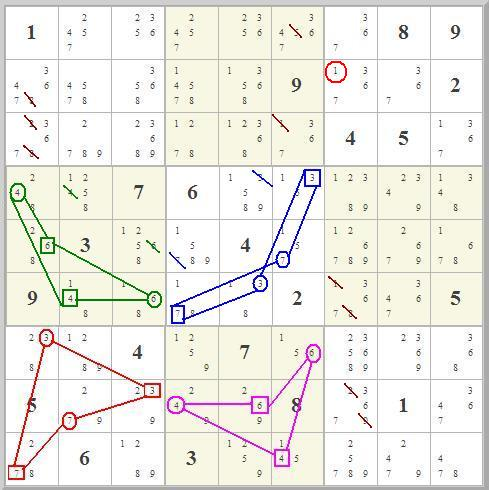But there's more! If you consider Row 8, our number chain pair analysis leads to the conclusion that both Blocks 7 and Blocks 8 contain a 29 pair in the second row. That amounts to a 29 naked pair in Row 8, and allows elmination of r8c7#2 and r8c7#9.

The same reasoning allows elimination of all of the following:
 2 and 8 from the first column of Block 1 1 and 5 from the third column of Block 2 1 and 8 from r6c7

And, best of all, this reveals a 367 naked triple in Column 7. We can set r2c7=1. Now we are getting somewhere.

### Medusa Strong Chain Pairing Analysis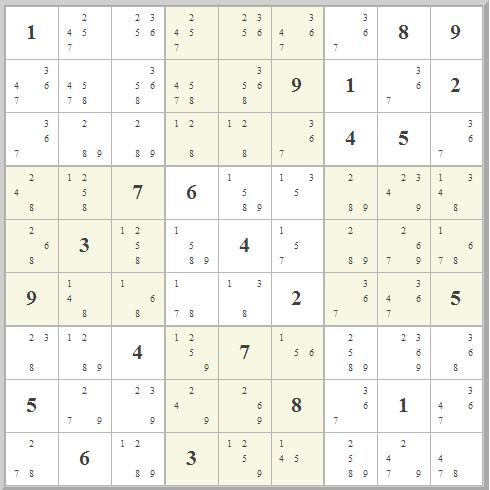With this first known value we get more simple eliminations and additional naked triples, leading finally to the configuration shown on the right.

The steps that break this puzzle include:
1. Showing that the two Medusa chains in Blocks 5 and 7 are linked.
2. Showing that because of this link, certain possibilities can be eliminated.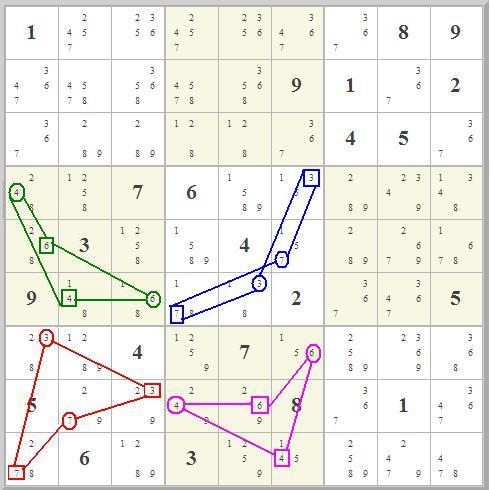Proving (1) is not difficult. What we are doing is determining whether the two 37 chains in Blocks 5 and 7 are in fact synchronized the way they are shown on the right (with r9c1=7 when r5c6<>7) or not. The proof is very simple. Consider the effect of r9c1=7 and r5c6=7:
• The two 7s at r9c1 and r5c6 conspire in Row 3 to force r3c9=7.
• This forces 7s into r8c7 and (especially) r6c8.
• That forces 4 out of r6c8 and into r6c2.
• The two 3s at r8c3 and r6c5 conspire in Column 7 to force r1c7=3.
• This forces 3s into r3c6 and (especially) r2c1.
• But that forces 4 out of r2c1 and into r4c1.
• It's impossible to have two 4s in a block.

OK, now consider the alternative for this chain syncing: r8c2=7 and r6c4=7. The analysis is identical! Once again, the two 7s conspire to force 4 into r6c2, and the two 3s conspire to force 4 into r4c1.

This proves that the two Medusa chains in Blocks 5 and 7 are synced as shown.

A similar though more complex analysis involving almost-locked sets concludes that blocks 4 and 8 are also linked as shown.

### End Game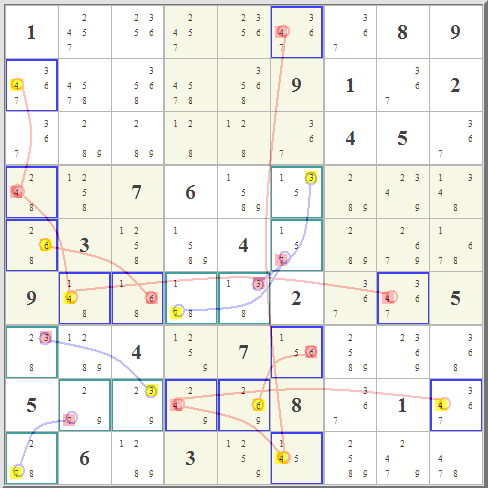At this point we have two main chains. It is a relatively simple matter to determine which polarity of the red chain is correct. We simply select red or yellow polarity and see what happens. Relatively straightforward checking, including almost-locked pair analysis proves that the correct polarity of this chain is the one marked in yellow. From this point on, the solution is straightforward, giving the solution to this puzzle.